Refer to our Texas Go Math Grade 1 Answer Key Pdf to score good marks in the exams. Test yourself by practicing the problems from Texas Go Math Grade 1 Unit 1 Assessment Answer Key.

Vocabulary

Circle the sum. (p.122)
Underline the differeñce. (p.166)

Question 1.
4 + 9 = 13The sum of 4 and 9 = 13

Question 2.
3 – 4 = 9
3 – 4 = 1

Concepts and Skills

Write how many.

Question 3.
How many bears? TEKS 1.3.B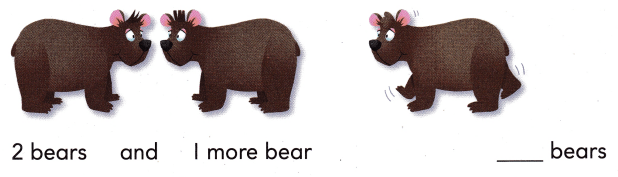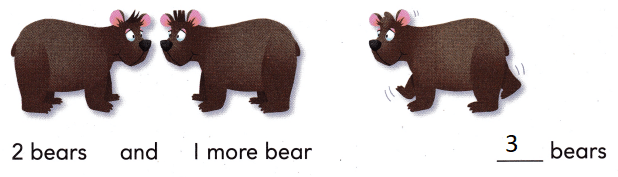Explanation:
There are 2 + 1 = 3 bears in all

Circle the part you are taking from, or separating from, the group. Then cross it out. Write how many there are now. TEKS 1.3.B

Question 4.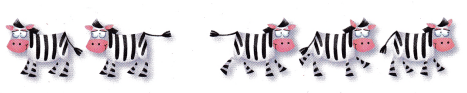There are 5 zebras.
3 zebras walk away.
How many zebras are there now?
__ zebras now.
2  zebras now.
Explanation:
5 – 3 = 2
The difference of 5 and 3 is 2

Texas Test Prep

Use. Choose the correct answer.

Question 5.
Which number does the model show? TEKS 1.2.B.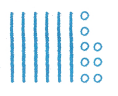(A) 86
(B) 68
(C) 18
Explanation:
The model shows 68
Line represents 10 and dots represent as 1

Question 6.
Which model shows the number? TEKS 1.2.C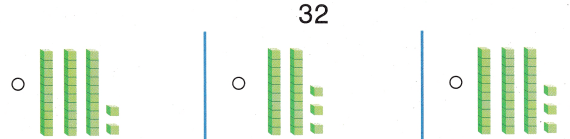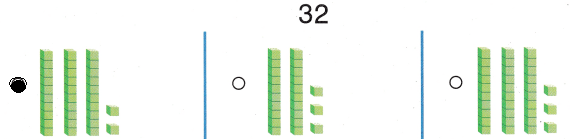Explanation:
The model shows 32
Line represents 10 and dots represent as 1
Bubbled the correct option

Question 7.
Which shows the model?(A) 100 + 2
(B) 100 + 20 + 2
(C) 100 + 10 + 2
Explanation:
The above base 10 blocks method shows 100 + 10 + 2 = 112

Question 8.
Which quick picture shows 110? TEKS 1.2.C.Explanation:
The above base 10 blocks method shows 100 + 10 + 1 = 111

Question 9.
Which number sentence does this model show? TEKS 1.3.A.(A) 5 + 5 = 10o
(B) 10 – 5 = 5
(C) 10 + 5 = 15
Explanation:
The ten frame shows
10 + 5 = 15

Texas Test Prep

Question 10.
Lee chooses a number > 72. Which shows a number she may choose? TEKS 1.2.G
(A) 75
(B) 15
(C) 69
Explanation:
75 shows the number that she may choose

Question 11.
Which of these is ¡n order from least to greatest? TEKS 1.2.F
(A) 23, 32, 90
(B) 75, 65, 85
(C) 54, 59, 50
Explanation:
23 , 32 , 90 are in order

Question 12.
Which number could belong at the dot? TEKS 1.2.F.(A) 78
(B) 88
(C) 68
Explanation:
The dot represents 78

Question 13.
Which shows how many? TEKS 1.3.B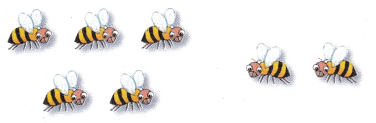(A) 6
(B) 3
(C) 7
Explanation:
There are 5 + 2 = 7 bees in all

Question 14
Which is a way to make 10? TEKS 1.3.C
(A) 4 + 5
(B) 6 + 2
(C) 4 + 6
Explanation:
The sum of 4 and 6 is 10

Texas Test Prep

Question 15.
What is the difference? TEKS 1.3.B, 1.5.E(A) 3
(B) 4
(C) 1
Explanation:The difference of 5 and 2 = 3

Question 16.
Selectto solve.

Jack has some marbles. He has ten more red marbles than blue marbles. He has fewer yellow marbles than blue marbles.
How many of each color marble could Jack have?

• What information am I given?
• What is my plan or strategy?
• How can I solve?
• How can I check my answer?